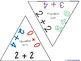# Addition - Equivalent Sums - Triangle GameSubject
Resource Type
File Type

PDF

(1 MB|65 pages)
Product Rating
Standards
• Product Description
• StandardsNEW
Addition - Equivalent Sums - Triangle Game is and engaging way to help students practice adding fluently and to help them memorize their basic facts.
To Play:
Pass out several playing cards to the students playing the game.
Lay one card on the table or floor.
Everyone checks their playing cards to see if they are holding a card that has an expression that has an equivalent sum.
If they have a card that has an equivalent sum they lay that card down as soon as they realize that they are holding the card.
There will be several cards that could work so more than one person could find an equivalent card. The first one to lay their card down gets to keep their card there.
This game is engaging because everyone is playing at the same time….there are no turns. Each player is required to constantly be looking at the opportunities on the table and looking for matches in their hand.

I also play by the rule that the expressions must be different while being equivalent.
Yes – 3+4 and 5+2 can be matched up, as well as 5 + 4 and 4 + 5
No – 3 + 2 and 3+2 are not used unless the game is stalled and the card is needed to continue playing.
I hope you and your students enjoy this resource and it helps your students grow.
Fluently add and subtract within 20 using mental strategies. By end of Grade 2, know from memory all sums of two one-digit numbers.
Understand the meaning of the equal sign, and determine if equations involving addition and subtraction are true or false. For example, which of the following equations are true and which are false? 6 = 6, 7 = 8 - 1, 5 + 2 = 2 + 5, 4 + 1 = 5 + 2.
Add and subtract within 20, demonstrating fluency for addition and subtraction within 10. Use strategies such as counting on; making ten (e.g., 8 + 6 = 8 + 2 + 4 = 10 + 4 = 14); decomposing a number leading to a ten (e.g., 13 - 4 = 13 - 3 - 1 = 10 - 1 = 9); using the relationship between addition and subtraction (e.g., knowing that 8 + 4 = 12, one knows 12 - 8 = 4); and creating equivalent but easier or known sums (e.g., adding 6 + 7 by creating the known equivalent 6 + 6 + 1 = 12 + 1 = 13).
Relate counting to addition and subtraction (e.g., by counting on 2 to add 2).
Apply properties of operations as strategies to add and subtract. If 8 + 3 = 11 is known, then 3 + 8 = 11 is also known. (Commutative property of addition.) To add 2 + 6 + 4, the second two numbers can be added to make a ten, so 2 + 6 + 4 = 2 + 10 = 12. (Associative property of addition.)
Total Pages
65 pages
N/A
Teaching Duration
Lifelong tool
Report this Resource to TpT
Reported resources will be reviewed by our team. Report this resource to let us know if this resource violates TpT’s content guidelines.